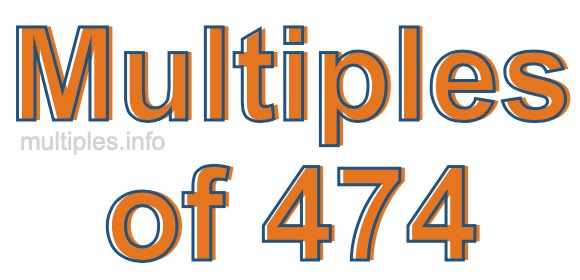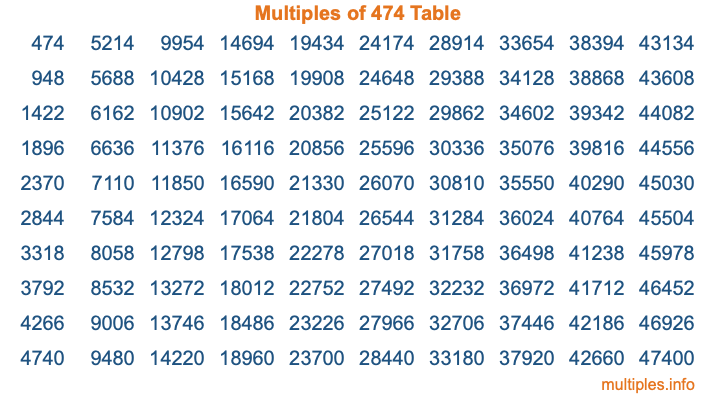Multiples of 474Welcome to the Multiples of 474 page. Here we will first teach you everything you will ever need to know about the multiples of 474, and then give you a study guide summary of everything we taught you to make sure you remember it all. Use this page to look up facts and learn information about the multiples of 474. This page will make you a multiples of four hundred seventy-four expert!

Definition of Multiples of 474
Multiples of 474 are all the numbers that when divided by 474 equal an integer. Each of the multiples of 474 are called a multiple. A multiple of 474 is created by multiplying 474 by an integer.

Therefore, to create a list of multiples of 474, you start with 1 multiplied by 474, then 2 multiplied by 474, then 3 multiplied by 474, and so on for as long as you want. Thus, the list of the first five multiples of 474 is 474, 948, 1422, 1896, and 2370. To see a larger list of multiples of 474, see the printable image of Multiples of 474 further down on this page. We also have a category where you can choose any nth multiple of 474.

Multiples of 474 Checker
The Multiples of 474 Checker below checks to see if any number of your choice is a multiple of 474. In other words, it checks to see if there is any number (integer) that when multiplied by 474 will equal your number. To do that, we divide your number by 474. If the the quotient is an integer, then your number is a multiple of 474.

Is  a multiple of 474?

Least Common Multiple of 474 and ...
A Least Common Multiple (LCM) is the lowest multiple that two or more numbers have in common. This is also called the smallest common multiple or lowest common multiple and is useful to know when you are adding our subtracting fractions. Enter one or more numbers below (474 is already entered) to find the LCM.

Check out our LCM Calculator if you need more details about the Least Common Multiple or if you need the LCM for different numbers for adding and subtraction fractions.

nth Multiple of 474
As we stated above, 474 is the first multiple of 474, 948 is the second multiple of 474, 1422 is the third multiple of 474, and so on. Enter a number below to find the nth multiple of 474.

th multiple of 474

Multiples of 474 vs Factors of 474
474 is a multiple of 474 and a factor of 474, but that is where the similarities end. All postive multiples of 474 are 474 or greater than 474. All positive factors of 474 are 474 or less than 474.

Below is the beginning list of multiples of 474 and the factors of 474 so you can compare:

Multiples of 474: 474, 948, 1422, 1896, 2370, etc.

Factors of 474: 1, 2, 3, 6, 79, 158, 237, 474

As you can see, the multiples of 474 are all the numbers that you can divide by 474 to get a whole number. The factors of 474, on the other hand, are all the whole numbers that you can multiply by another whole number to get 474.

It's also interesting to note that if a number (x) is a factor of 474, then 474 will also be a multiple of that number (x).

Multiples of 474 vs Divisors of 474
The divisors of 474 are all the integers that 474 can be divided by evenly. Below is a list of the divisors of 474.

Divisors of 474: 1, 2, 3, 6, 79, 158, 237, 474

The interesting thing to note here is that if you take any multiple of 474 and divide it by a divisor of 474, you will see that the quotient is an integer.

Multiples of 474 Table
Below is an image of the first 100 multiples of 474 in a table. The table is in chronological order, column by column. The first column has the first ten multiples of 474, the second column has the next ten multiples of 474, and so on.The Multiples of 474 Table is also referred to as the 474 Times Table or Times Table of 474. You are welcome to print out our table for your studies.

Negative Multiples of 474
Although not often discussed or needed in math, it is worth mentioning that you can make a list of negative multiples of 474 by multiplying 474 by -1, then by -2, then by -3, and so on, to get the following list of negative multiples of 474:

-474, -948, -1422, -1896, -2370, etc.

Multiples of 474 Summary
Below is a summary of important Multiples of 474 facts that we have discussed on this page. To retain the knowledge on this page, we recommend that you read through the summary and explain to yourself or a study partner why they hold true.

There are an infinite number of multiples of 474.

A multiple of 474 divided by 474 will equal a whole number.

474 divided by a factor of 474 equals a divisor of 474.

The nth multiple of 474 is n times 474.

The largest factor of 474 is equal to the first positive multiple of 474.

474 is a multiple of every factor of 474.

474 is a multiple of 474.

A multiple of 474 divided by a divisor of 474 equals an integer.

474 divided by a divisor of 474 equals a factor of 474.

Any integer times 474 will equal a multiple of 474.

Multiples of a Number
Here you can get the multiples of another number, all with the same attention to detail as we did for multiples of 474 on this page.

Multiples of
Multiples of 475
Did you find our page about multiples of four hundred seventy-four educational? Do you want more knowledge? Check out the multiples of the next number on our list!